### IMO Shortlist 2005 problem N5

Kvaliteta:
Avg: 0.0
Težina:
Avg: 8.0
Denote by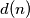$d(n)$ the number of divisors of the positive integer$n$. A positive integer$n$ is called highly divisible if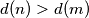$d(n) > d(m)$ for all positive integers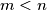$m < n$.
Two highly divisible integers$m$ and$n$ with$m < n$ are called consecutive if there exists no highly divisible integer$s$ satisfying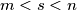$m < s < n$.

(a) Show that there are only finitely many pairs of consecutive highly divisible
integers of the form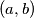$(a, b)$ with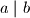$a\mid b$.

(b) Show that for every prime number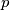$p$ there exist infinitely many positive highly divisible integers$r$ such that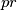$pr$ is also highly divisible.
Source: Međunarodna matematička olimpijada, shortlist 2005